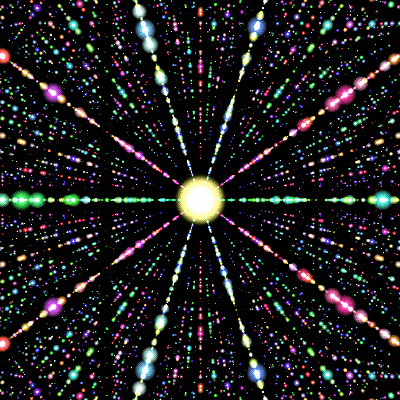This demo requires HTML5 Canvas support.

## Help

What am I seeing here?

• Turn: arrow keys and <,, >. or mouse/finger dragging.
• Fly: spacebar and Z or press-and-hold.
• Zoom: + and -, mouse scrolling, or pinch zoom. (Hint: lock onto a point first.)
• Lock on to a point: double-tap.
• Toggle first-person view: triple-tap.

Code written by Pteromys and released under the Unlicense.

This page draws the icosahedral 3-D quasicrystal: a thin 3-D slice of a 6-D lattice with icosahedral symmetry. Points are sized by their proximity to the slice and colored by how far they extend into the 3 unrepresented dimensions (red, green, and blue).

This quasicrystal has two curious properties:

1. It's the only 3-D lattice slice which is truly 3-D—all others with any symmetry merely repeat a 2-D pattern.
2. It's self-similar—a zoomed-in view is indistinguishable from a zoomed-out view.

## Self-similarity

Zooming in expands space by some factor $$s$$. To compensate for the spreading out of dots, we'll make more dots touch our slice by scaling the unseen 3 dimensions by $$\frac{1}{s}$$ (to keep the number of dots drawn roughly constant).

If $$s = 2 + \sqrt{5}$$, a miracle occurs: all dots land on other dots, and it's as if we never zoomed in. To understand why, we need to describe the 6-D lattice in more detail.

Let $$\zeta$$ be the 5th root of unity $$e^{2\pi i/5}$$. In $$\mathbf{R} \times \mathbf{C}$$, the points $\pm(\sqrt{5}, 0), \pm(1, 2\zeta^k)$ ($$k = 0$$ to $$4$$) are the twelve vertices of an icosahedron, and their sums are the dots we see. The unseen 3 dimensions are a second set of $$\mathbf{R} \times \mathbf{C}$$ coordinates that replace $$\zeta$$ with the algebraically indistinguishable $$\zeta^2$$. As $$\sqrt{5} = 1 + 2(\zeta + \zeta^{-1})$$, this also ends up replacing $$\sqrt{5}$$ by $$-\sqrt{5}$$. You can check using dot products that the twelve vertices now point in six perpendicular directions in the 6-D combined coordinate system.

Scaling by $$2 + \sqrt{5}$$ in the first two coordinates amounts to replacing each vertex with the sum of twice itself and its five neighbors—thus taking dots to other dots. In the last two coordinates, this becomes scaling by $$2 - \sqrt{5}$$, and the miracle stems from the fact that $(2 + \sqrt{5})(2 - \sqrt{5}) = -1 .$ That is, this scaling is what we'd obtain with a zoom factor of $$2 + \sqrt{5}$$.

This also occurs in the 2-D quasicrystal with 5-fold symmetry, for which a related phenomenon is illustrated by the game Lucy and Lily.

## Uniqueness

What we're looking for is a group of symmetries $$G$$ of a higher-dimensional lattice $$\mathbf{Z}^n \subset \mathbf{R}^n$$, along with a 3-plane $$V \subset \mathbf{R}^n$$ (the slice) on which $$G$$ acts irreducibly (the non-2-D condition, which amounts to not having an axis).

Of the seven non-axial point groups in 3 dimensions, only the two icosahedral groups don't already preserve an ordinary 3-D lattice. These are the alternating group $$A_5$$ and the product $$A_5 \times \{\pm 1\}$$; so it suffices to find a list of representations of $$A_5$$ over integers and real numbers.

(One detail remains: $$A_5$$ might only be the quotient of $$G$$ by a normal subgroup $$N$$. Fortunately, the map $x \mapsto \sum_{g \in N} gx$ is an endomorphism of $$\mathbf{Z}^n$$ on whose image $$A_5$$ acts.)